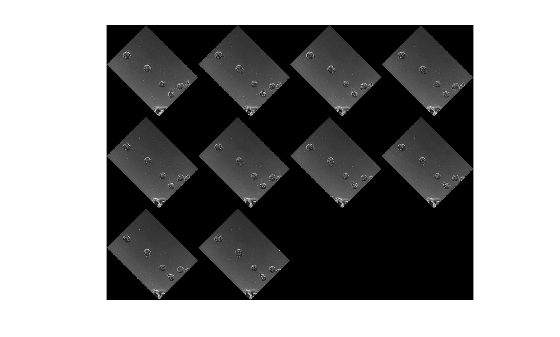# Warper

Apply same geometric transformation to many images efficiently

## Description

A `Warper` object applies an `affine2d` or `projective2d` geometric transformation to images with a specific size.

## Creation

### Syntax

``w = images.geotrans.Warper(tform,inputSize)``
``w = images.geotrans.Warper(tform,inputRef)``
``w = images.geotrans.Warper(tform,inputRef,outputRef)``
``w = images.geotrans.Warper(sourceX,sourceY)``
``w = images.geotrans.Warper(___,Name,Value)``

### Description

example

````w = images.geotrans.Warper(tform,inputSize)` creates an image warper from the geometric transformation object `tform` and sets the InputSize property.```
````w = images.geotrans.Warper(tform,inputRef)` specifies the coordinate system of the input images, `inputRef`.```
````w = images.geotrans.Warper(tform,inputRef,outputRef)` specifies the coordinate system of the output image, `outputRef`. This syntax can be used to improve performance by limiting the application of the geometric transformation to a specific output region of interest.```
````w = images.geotrans.Warper(sourceX,sourceY)` specifies the input image coordinates, `sourceX` and `sourceY`, required to perform the geometric transformation.```
````w = images.geotrans.Warper(___,Name,Value)` sets the Interpolation and FillValue properties using one or more name-value pair arguments. Enclose each property name in single quotes.For example, ```warper = images.geotrans.Warper(tform,size(im),'FillValue',1)``` specifies a fill value of `1` for pixels outside the original image.```

### Input Arguments

expand all

Geometric transformation, specified as an `affine2d` or `projective2d` geometric transformation object.

Referencing object associated with the input image, specified as an `imref2d` spatial referencing object.

Referencing object associated with the output image, specified as an `imref2d` spatial referencing object.

Input image coordinates, specified as a 2-D matrix the same size as the required output image. Each (x, y) index in `sourceX` and `sourceY` specifies the location in the input image for the corresponding output pixel.

Data Types: `single`

## Properties

expand all

Size of the input images, specified as a 2- or 3-element vector of positive integers.

Size of the first two dimensions of the output image, specified as a 2-element vector of positive integers.

Interpolation method, specified as `'linear'`, `'nearest'`, or `'cubic'`.

Data Types: `char` | `string`

Value used for output pixels outside the input image boundaries, specified as a numeric scalar. `Warper` casts the fill value to the data type of the input image.

## Object Functions

 `warp` Apply geometric transformation

## Examples

collapse all

Pick a set of images of the same size. The example uses a set of images that show cells.

`imds = imageDatastore(fullfile(matlabroot,'toolbox','images','imdata','AT*'));`

Create a geometric transform to rotate each image by 45 degrees and to shrink each image.

```tform = affine2d([ 0.5*cos(pi/4) sin(pi/4) 0; -sin(pi/4) 0.5*cos(pi/4) 0; 0 0 1]);```

Create a `Warper` object, specifying the geometric transformation object, `tform`, and the size of the input images.

```im = readimage(imds,1); warper = images.geotrans.Warper(tform,size(im));```

Determine the number of images to be processed and preallocate the output array.

```numFiles = numel(imds.Files); imr = zeros([warper.OutputSize 1 numFiles],'like',im);```

Apply the geometric transformation to each of the input images by calling the `warp` function of the `Warper` object.

```for ind = 1:numFiles im = read(imds); imr(:,:,1,ind) = warp(warper,im); end```

Visualize the output images. (Turn off the warning message about the images being scaled for display.)

```warning('off','images:initSize:adjustingMag') montage(imr);```## Tips

• If the input images are RGB images or 3-D grayscale images of size m-by-n-by-p, then `warp` applies the transformation to each color channel or plane p independently.

## Algorithms

`Warper` is optimized to apply the same geometric transformation across a batch of same size images. `Warper` achieves this optimization by splitting the warping process into two steps: computation of the transformed coordinates (done once) and interpolation on the image (done for each image). Compared to `imwarp`, this approach speeds up the whole process significantly for small to medium-sized images, with diminishing returns for larger images.

### Objects

Introduced in R2017b